###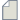Author Topic: Transmission as function of electrode Fermi level  (Read 366 times)

0 Members and 1 Guest are viewing this topic.

####MolFET93

• Regular QuantumATK user
•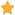• Posts: 5
• Country: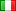• Reputation: 0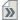##### Transmission as function of electrode Fermi level
« on: March 15, 2023, 19:45 »
Dear QuantumATK community,

I would like to support my single molecule STM-BJ data with DFT calculations.
I got a certain value of conductance at break-off distance from STM-BJ measurements, this should correspond to the fully streatched molecule between the two electrodes (substrate and tip).
I run DFT+NEGF simulation (GGA, PBE, DZP and SZP for Au atoms) and I compared the experimental conductance value with the transmission value at the fermi level (0 eV)  multiplied by the quantum conductance G0 (G=G0*T(Ef)), Ef fermi level).
The theoretical conductance G and the experimental one differ of order of magnitude.
This is related to different experimental configuration of electrodes w.r.t. the ideal device configuration for the theoretical calculation, and thus also to different electrode fermi levels w.r.t. the ones computed by the LCAO calculator.

So, I would like to compute only the transmission value at the fermi level (T(0 eV)) by varying the value of electrode fermi level w.r.t. the one obtained with QuantumATK, to understand approximately which value of electrode fermi level provides a good match with the experimental conductance value.

How I can force the value of the electrode fermi level in the transmission calculation starting from a DeviceConfiguration already obtained with LCAO calculator?

Thank you.
Best wishes

####Anders Blom

• QuantumATK Staff
• Supreme QuantumATK Wizard
•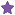• Posts: 5287
• Country:• Reputation: 88##### Re: Transmission as function of electrode Fermi level
« Reply #1 on: March 30, 2023, 21:27 »
Doesn't the transmission spectrum T(E) correspond to precisely what you are asking? Otherwise, what is the physics behind changing the Fermi level of one of the electrodes? Normally you do this with a bias (i.e. voltage difference), so you might also just compute T(E=0) for finite bias. But if the voltage is the same on both electrodes, nothing happens, it's just a shift of the overall energy zero-level.